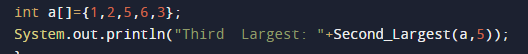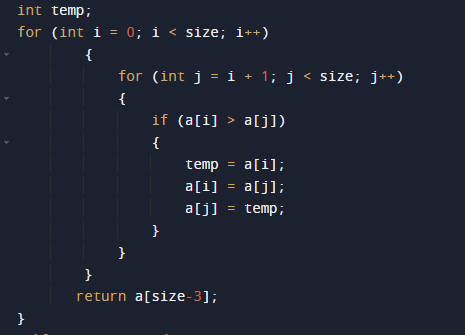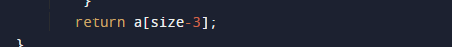# Java Program to Find 3rd Largest Number in an array

In this tutorial you will learn about the Java Program to Find 3rd Largest Number in an array and its application with practical example.

In this tutorial, we will learn to create a Java Program to Find 3rd Largest Number in an array using java programming.

## Prerequisites

Before starting with this tutorial, we assume that you are best aware of the following Java programming concepts:

• Java Operators.
• Basic Input and Output function in Java.
• Class and Object in Java.
• Basic Java programming.
• If-else statements in Java.
• For loop in Java.

## Java Program to Find 3rd Largest Number in an array

In this program we will find smallest element in an array using nested for loop. We would first declared and initialized the required variables. Next, we will find smallest number in an array. Lets have a look at the code.

## Output

3rd Largest in an array[].In the above program, we have first declared and initialized a set variables required in the program.

• a[]= it will hold array values
• l=  it will hold length of an array.
• i and j for iteration
• temp = it will hold temporary value of an array.

After declaring variables we initiate values in  an array[]here we will call a function where we fin second greatest in an array[].## 3rd Largest in an array

• Compare the first two elements of the array
• If the first element is greater than the second exchange them.
• Then, compare 2nd and 3rd elements if the second element is greater than the 3rd swap them.
• Repeat this till the end of the array.
• and at last function return a[size-3];
• which is the second largest in an array[].and finally we will print the result it means the second largest in an  array[].In this tutorial we have learn about the Java Program to Find 3rd Largest Number in an array and its application with practical example. I hope you will like this tutorial.